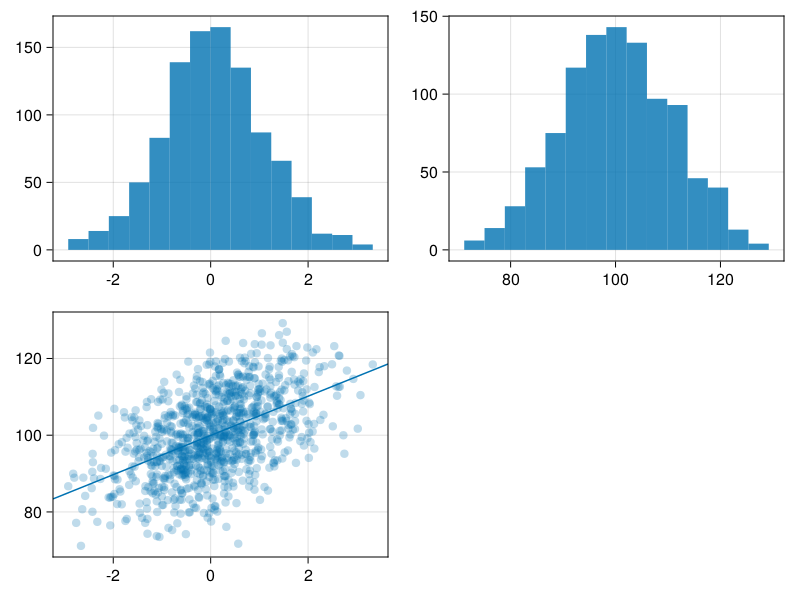# Tutorial

using Faux # for data simulation (under development!)
using DataFrames # for data wrangling
using CairoMakie # for plots
using GLM # to calculate regression lines (why doesn't Makie do this?)
using Statistics
import Random

rng = Random.seed!(8675309); # make randomness predictable :)
Random.TaskLocalRNG()

## Simulate by Design

By default, sim_design() gives you a data frame with n = 100 observations of a single normally distributed variable called y, with mean = 0 and sd = 1. Here, we will simulate smaller n to make tables easier to see.

df = sim_design(n = 5)
5×2 DataFrame
Rowidy
StringFloat64
1id10.70632
2id2-0.366571
3id3-0.180916
4id4-0.684639
5id5-0.275842

You can change the name of the dependent variable or the id column.

df = sim_design(n = 5, dv = "score", id = "subj")
5×2 DataFrame
Rowsubjscore
StringFloat64
1subj10.46199
2subj20.527764
3subj31.66803
4subj4-0.112542
5subj50.421035

b = ["pet" => ["dog", "cat"]]
df = sim_design(n = 3, between = b)
6×3 DataFrame
Rowidpety
StringSubStrin…Float64
1id1dog-1.10909
2id2dog0.167813
3id3dog-0.623059
4id4cat0.0341544
5id5cat1.23148
6id6cat-0.480946

And within-subject variables.

w = ["cond" => ["ctl", "exp"]]
df = sim_design(n = 3, within = w)
3×3 DataFrame
Rowidctlexp
StringFloat64Float64
1id1-0.00787680.310653
2id21.04838-1.33857
3id30.9378781.34539

You can return a long version of your data.

df = sim_design(n = 3, within = w, long = true)
6×3 DataFrame
Rowidcondy
StringSubStrin…Float64
1id1ctl0.322081
2id2ctl1.01367
3id3ctl-0.526296
4id1exp-0.552148
5id2exp-0.625933
6id3exp0.890368

Set the means, standard deviations, and correlations. Set empirical = true to set the sample parameters, rather than the population parameters.

b = ["pet" => ["dog", "cat"]]
w = ["cond" => ["ctl", "exp1", "exp2"]]
mu = Dict("dog" => Dict("ctl" => 10, "exp1" => 20, "exp2" => 30),
"cat" => Dict("ctl" => 40, "exp1" => 50, "exp2" => 60))
sd = Dict("cat" => Dict("exp1" => 5, "exp2" => 6, "ctl" => 4),
"dog" => Dict("ctl" => 1, "exp1" => 2, "exp2" => 3))
r = Dict("dog" => [.1, .2, .3],
"cat" => [.4, .5, .6])

df = sim_design(n = 5, within = w, between = b,
mu = mu, sd = sd, r = r,
empirical = true)
10×5 DataFrame
Rowidpetctlexp1exp2
StringSubStrin…Float64Float64Float64
1id01dog9.4998117.146627.2636
2id02dog9.7778122.033526.7213
3id03dog8.7665320.846533.002
4id04dog11.342821.212632.9576
5id05dog10.61318.760830.0555
6id06cat40.651552.952559.6213
7id07cat44.714254.705361.7551
8id08cat42.792947.134565.6898
9id09cat35.553552.576162.9258
10id10cat36.287942.631550.0079
dogs = filter(row -> row.pet == "dog", df)
get_params(dogs)
3×6 DataFrame
Rowvarctlexp1exp2meansd
StringFloat64Float64Float64Float64Float64
1ctl1.00.10.210.01.0
2exp10.11.00.320.02.0
3exp20.20.31.030.03.0
cats = filter(row -> row.pet == "cat", df)
get_params(cats)
3×6 DataFrame
Rowvarctlexp1exp2meansd
StringFloat64Float64Float64Float64Float64
1ctl1.00.40.540.04.0
2exp10.41.00.650.05.0
3exp20.50.61.060.06.0
df = sim_design(n = 1000,
within = ["axis" => ["x", "y"]],
mu = [0, 100],
sd = [1, 10],
r = 0.5);

# ugh, I want ggplot2 :(
f = Figure()
hist(f[1,1], df.x)
hist(f[1,2], df.y)
scatter(f[2,1],df.x, df.y, alpha = 0.25)
m = GLM.lm(@formula(y ~ x), df)
ablines!(f[2,1], coef(m)...)
f## Repeats

Add the rep argument to simulate multiple repeats.

df = sim_design(between = ["cond" => ["ctl", "exp"]],
mu = [0, 0.25], rep = 1000, long = true)

Use a split-apply-combine pattern to run an analysis. First, define an analyss fucntion that takes a data frame as the only argument, and returns a data frame of analysis values.

# define an analysis function
function analysis(df)
m = lm(@formula(y ~ cond), df)
stats = coeftable(m)
return DataFrame(stats)
end

# test the analysis function
analysis(df)
2×7 DataFrame
RowNameCoef.Std. ErrortPr(>|t|)Lower 95%Upper 95%
StringFloat64Float64Float64Float64Float64Float64
1(Intercept)0.0014440.003158730.4571440.647568-0.004747040.00763503
2cond: exp0.247970.0044671255.50990.00.2392140.256725

Split the data by rep, run the analysis on each rep, and calculate summary stats like mean coefficient or power.

# split the data by rep
df_grp = groupby(df, :rep)

# run the analysis on each rep
analyses = combine(df_grp, analysis)

# calculate summary stats by factor
combine(groupby(analyses, :Name),
x -> (
power = mean(x[!, 6] .< 0.05),
mean_coef = mean(x[!, 3]),
sd_coef = std(x[!, 3])
)
)
2×4 DataFrame
RowNamepowermean_coefsd_coef
StringFloat64Float64Float64
1(Intercept)0.0470.0014440.0973973
2cond: exp0.4110.247970.141287